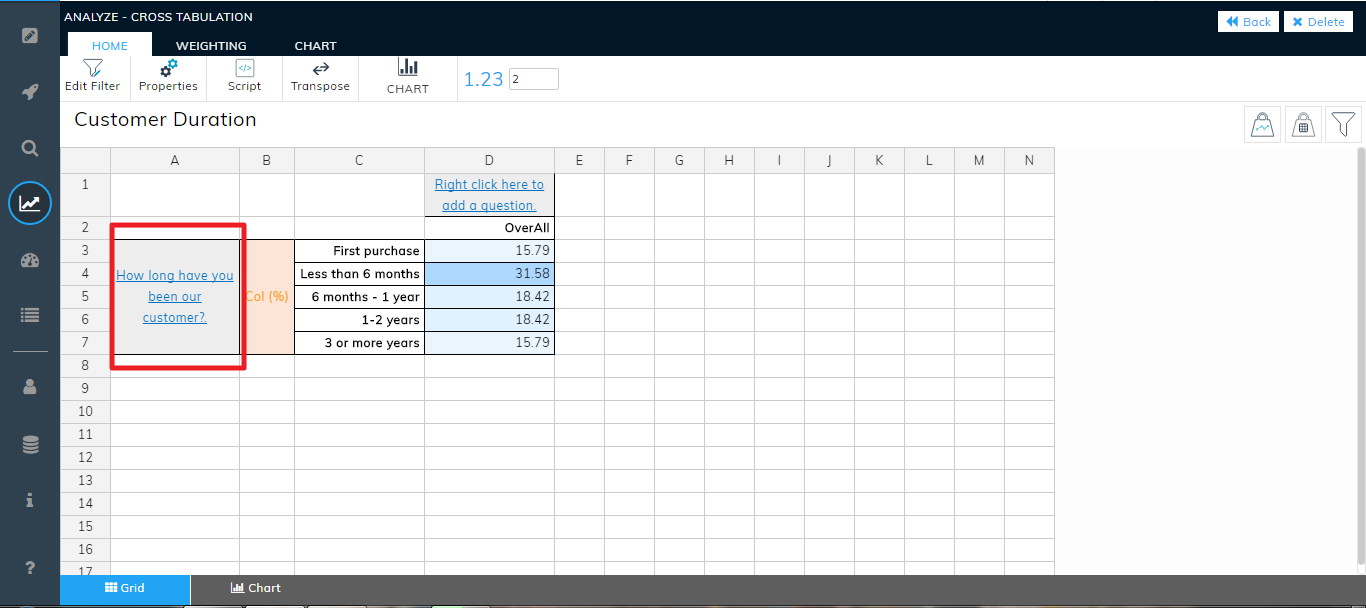In this article we will explain about the most common terminologies used for a table.

Side break

The variables in the row of a table are known as the side breaks. In the below example, 'How long have you been our customer?' is the side break.Top Break

The variables in the column of a table are known as the top breaks. In the below example, 'Created On' is the top break.Axis

It is defined as a fixed reference line for the measurement of coordinates. The 'Side Break' is the Y-axis and 'Top Break' is X-axis.

In the below example, NPS Trend is the side break and Y-axis variable. 'CreatedOn' is the top break and X-axis variable.